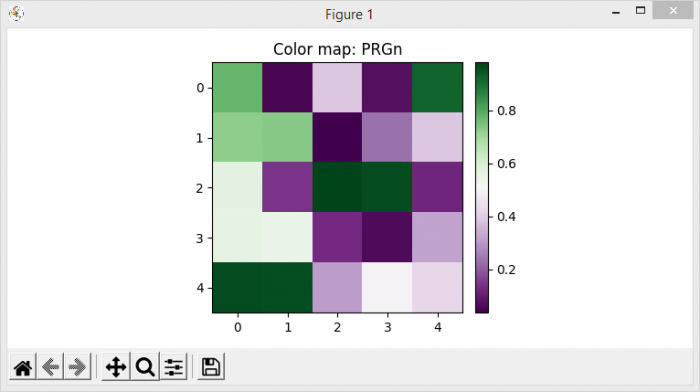# How to view all colormaps available in Matplotlib?

To view all colormaps available in Matplotlib, we can take the following steps −

• Set the figure size and adjust the padding between and around the subplots.
• Create a new figure or activate an existing figure.
• Add an '~.axes.Axes' to the figure as part of a subplot arrangement
• Make an axis that is divider on the existing axes.
• Create random data using numpy.
• Display the data as an image, i.e., on a 2D regular raster.
• Create a colorbar for a ScalarMappable instance, im.
• Set a title for the current figure.
• Animate the image with all colormaps available in matplotlib.
• Make an animation by repeatedly calling a function.
• To display the figure, use show() method.

## Example

import numpy as np
import matplotlib.pyplot as plt
import matplotlib.animation as animation
from mpl_toolkits.axes_grid1 import make_axes_locatable

plt.rcParams["figure.figsize"] = [7.50, 3.50]
plt.rcParams["figure.autolayout"] = True

fig = plt.figure()
div = make_axes_locatable(ax)
cax = div.append_axes('right', '5%', '5%')
data = np.random.rand(5, 5)
im = ax.imshow(data)
cb = fig.colorbar(im, cax=cax)
cmap = plt.colormaps()
tx = ax.set_title('Color map: {0}'.format(cmap))

def animate(i):
cax.cla()
data = np.random.rand(5, 5)
im = ax.imshow(data, cmap=cmap[i])
fig.colorbar(im, cax=cax)
tx.set_text('Color map: {0}'.format(cmap[i]))

ani = animation.FuncAnimation(fig, animate, frames=166)

plt.show()

## Output